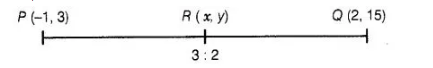# Find the coordinates of the point

Question:

Find the coordinates of the point $R$ on the line segment joining the points $P(-1,3)$ and $Q(2,5)$ such that $P R=\frac{3}{5} P Q$.

Solution:

According to the question,Given that, $P R=\frac{3}{5} P Q$

$\Rightarrow$ $P R=\frac{3}{5} P Q$

$\Rightarrow$ $\frac{P R+R Q}{P R}=\frac{5}{3}$

$\Rightarrow$ $1+\frac{R Q}{P R}=\frac{5}{3}$

$\Rightarrow$ $\frac{P Q}{P R}=\frac{5}{3}-1=\frac{2}{3}$

$\therefore$ $R Q: P R=2: 3$

or $P R: R Q=3: 2$

Suppose, $R(x, y)$ be the point which divides the line segment joining the points $P(-1,3)$ and $Q(2,5)$ in the ratio $3: 2$.

$\therefore$ $(x, y)=\left\{\frac{3(2)+2(-1)}{3+2}, \frac{3(5)+2(3)}{3+2}\right\}$

$\left[\because\right.$ by internal section formula, $\left.\left\{\frac{m_{2} x_{1}+m_{1} x_{2}}{m_{1}+m_{2}}, \frac{m_{2} y_{1}+m_{1} y_{2}}{m_{1}+m_{2}}\right\}\right]$

$=\left(\frac{6-2}{5}, \frac{15+6}{5}\right)=\left(\frac{4}{5}, \frac{21}{5}\right)$

Hence, the required coordinates of the point $R$ is $\left(\frac{4}{5}, \frac{21}{5}\right)$.